# Arithmetic - math problems

#### Number of problems found: 2530

• Comparing exponents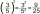A) 8 (6²)(8²) b) 16 (6²)(8²) c) (6¹+²) (8²)
• Supplementary angles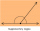(a + 30)° and (2a)° are the measure of two supplementary angles. Find the value of 'a'.
• Simplest form 3What fraction is 15 of 35 in simplest form?
• A burger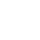The water starts with 41 1/4 cups of water how much water is left after ten 3 5/8 Scoops of water are removed?
• Licorice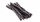A piece of licorice is 2/3 yard long.  If it is cut into 10 equal pieces, how long will each piece of licorice be?Add this two mixed numbers: 1 5/6 + 2 2/11=
• Divide 13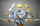Divide. Simplify your answer and write as an improper fraction or whole number. 14÷8/3
• Evaluate fractionsThe difference  of 5 1/2 and 2/3 is added to the product of 5/6 and 1/2The weight of the empty fruit basket is ¾ kg. We added ½ kg apples and 5/4 kg guavas. What will be the total weight of the fruit basket?
• The sum 13The sum of the exponents of the prime factors in the prime factorization of 196 is. .. .
• The rope 2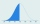The rope in the school gym is 10 ½ feet long. To make it easier to climb, Coach Hill tied a knot in the rope every ¾ foot. How many knots are in the rope?
• A science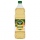A science experiment calls for making 3 and 2/3 cups of distilled water with 1 and 3/5 cups of vinegar and 2/3 cups of liquid detergent how much liquid in all and cups is needed?
• How many 16How many three-tenths are there in two and one-fourths?
• English and math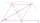1/5 of a class study English, and 3/5 study mathematics. If the class consist of 80 students, how many students study English and mathematics in the class?
• Fraction expressionWhich expression is equivalent to : minus 9 minus left parenthesis minus 4 start fraction 1 divided by 3 end fraction right parenthesis
• Sum and productThe sum of the real numbers x and y is 12. Their difference is 8. What is the value of xy?
• Cutting wireIf you cut a 3 ½ ft length wire into pieces that are 2 inches long, how many pieces of wire will you have?
• Kilos of meat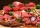Marry bought 6 1/3 kg of meat. She used 2 1/4 kg for Anita 2 1/8 kg for Maros and the rest for Adam. How many kilograms of meat did she used for Adam?
• Sign of a expressionWhat is the sign of expression: minus 18 start fraction 9 divided by 17 end fraction plus left parenthesis minus 18 start fraction 9 divided by 17 end fraction right parenthesis?
• SixteenSixteen minus the quotient of 12 and what number is thirteen?

Do you have an exciting math question or word problem that you can't solve? Ask a question or post a math problem, and we can try to solve it.

We will send a solution to your e-mail address. Solved examples are also published here. Please enter the e-mail correctly and check whether you don't have a full mailbox.

Please do not submit problems from current active competitions such as Mathematical Olympiad, correspondence seminars etc...